# A practical Guide to Datasist in Python

Datasist for data analysis and visualization.

Datasist is an open source python library that offers functions and methods for easy data analysis, visualization and also effectively structuring and managing data science projects.

In this article, I’m going to introduce you to the world of data analysis, data visualization and interpretation using python library called “Datasist”.

# Table of content

1. Intalling Datasist
2. Working with Datasist structdata
3. Feature engineering with Datasist
4. Data visualization with Datasist

Without wasting much time, Let’s get our legs wet on the practical guide to Datasist.

Installing Datasist

Datasist is available as a python package for linux, MacOS, Windows and can be intalled like any other python package.

If you have an existing Python environment activated, you can install datasist with the following command:

To install datasist in a virtual environment, you can use Anaconda package.

Note: You must have Anaconda installed.

To confirm that you already have Anaconda installed. You need to use the following command:

Now head on to creating a new virtual environment and install the latest version of Python. Let’s say Python 3.5 and above. You will use the following command:

The next step is to your environment. You will use the following command:

Now install datasist with the following command:

The next step is to test your installation using the following command:

Now that we are done with the installation. Let’s head to the next agenda on the list.

Working with datasist structdata

You ever imagine. What is Datasist?. Well, I will go by saying: Datasist is a python library that makes easy data analysis,visualization,cleaning,preparation for data scientist during prototyping.

Note: We will be using Jupyter notebook and we will be working with Data science Nigeria 2020 hackhaton dataset. After getting all this ready. Let’s dive into action.

Finally, open your jupyter notebook, import your libraries and dataset as shown below.

The structdata module has many functions for working with structured data in the Pandas DataFrame format. So, you can easily manipulate and analyze DataFrames. Let’s dive into the functions available.

1. Describe : We all know that Pandas also has describe function. Let’s take a look at Datasist describe method.

Lol, The datastruct module displays the following output:

(a) First five data points.

(b) Random five data points.

(c ) Last five data points

(d ) Shape of data set , Size of data set

(e ) Data types

(f ) Numerical features in the dataset

(g ) Categorical features in the dataset

(h ) Statistical Description of Columns

(i ) Description of Categorical features

(j ) Unique class count of Categorical features

(k ) Missing values in data

Isn’t it amazing?. Lets’s get to another aspect of datastruct module function.

2. check_train_test_set: To use this function,()train_df and test_df) function must be passed. A common index(Applicant_ID) and any feature availabel in both dataset. Let’s have a look.

Now, let’s see our output:

3. display_missing values: You can check for the missing values in the dataset. Let’s check it out.

Let’s see what the output gives us:

It’s amazing right?

4. get_cats_feats and get_num_feats : This functions are used to retrieve categorical and numerical features and they give their output as a list.

Let’s work with the function and see what it looks like:

You see that, right?. Let’s check the numerical features.

This looks interesting and seems easy.

5. get_unique_counts : In a categorical features, you can use the function to get the unique class. Let’s have a look below.

You see how that works? huh? pretty easy and straightforward.

6. join_train_test : You can use the function(join_train_and_test) function to concatenate both the train and test set. Let’s do some stuffs:

The structdata module in datasist also has more functions. You can head to datasist API documentation to learn more.

Feature engineering with datasist

Feature engineering is the process of using data’s domain knowledge to create features that make machine learning algorithm works. It’s the act of extracting important features from raw data and transforming them into formats that are suitable for machine learning.

Now, let’s explore some of the feature_engineering module of datasist.

1. drop_missing: The functions drops feature with missing values. Let’s take a look below:

Let’s see how the output goes:

2. drop_redundant : This functions removes features with no variance. Let’s create a new dataset. Let’s have a look:

Let’s see what the output looks like:

Now, check the dataset. You see that it is redundant which means that it has the same class all through. We will drop the column by using this function. Check below:

The output goes thus:

Ooops, cool. You see that?

3. convert_dtypes : This function takes DataFrame automatically features that are not represented on their right types. Let’s have a look:

The output gives:

Now, let’s look at data types.

Note: The features of Age is supposed to be integer. By using convert_dtype function. It is automatically fixed. Let’s have a look:

4. fill_missing_cats : This function automatically fills in the missing values. Let’s check:

5. fill_missing_values : This function works on numerical features and you can specify a fill strategy (mean, median and mode). Let’s check:

Cool, you see that? Pretty easy. Let’s dive into the next one which is Visualization with datasist.

## Visualization with datasist

Before diving into action, let’s re-import the dataset we previously used.

The output goes thus:

Now, we will be dividing our visualization into two parts namely:

(a) Visualization for categorical features

(b) Visualization for numerical features

Visualization for categorical features

Visualization for the categorical features include: violinplot, countplot,boxplot etc. Now, let’s start getting our hands on them one after the other.

• countplot : This makes a barplot of all the categorical features to show their class count. Let’s check:

The output goes thus:

• boxplot : This makes a box plot of all numerical features against a categorical target column. Have a look:
• catbox : This is used to make a side by side bar plot of all categorical features in a dataset against a categorical target. Let’s check:

Let’s see the output:

Visualization for numerical features

Visualization for numerical features include plots like: scatterplot, histogram,kde plots etc. Let’s dive into action:

• plot_missing : This function can be used to visualize the missing values in a dataset. Let’s have a look:

Now, let’s see the output:

Now, we are done with the tutorials. Now, you will be able to use datasist for problems for data analysis and data visualization like(pandas, seaborn, matplotlib and many more tools).

Note: The notebook for this tutorial is available here.

--

--

--

## More from Analytics Vidhya

Analytics Vidhya is a community of Analytics and Data Science professionals. We are building the next-gen data science ecosystem https://www.analyticsvidhya.com

## Virtual Background for Video Conferencing using Machine Learning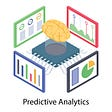## What I Learned Coding Google From Scratch## What is Customer lifetime value?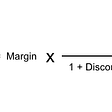## R: What Is Logistic Regression? How Can I Program It?## Interested In Data Science…!!But Don’t Know Where Do You Fit In This Data-Driven Career..???## The Strange Story of the Paxos AlgorithmHi all👋. I’m a data scientist |Machine learning engineer |Researcher| CS undergrads @EKSUUNI

## House Price Prediction using Machine Learning with Python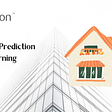## Housing Bubbles in History — A Detection Using Python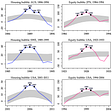## What is Agglomerative clustering and how to use it with Python Scikit-learn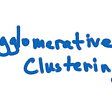## Create your own dataset in pandas: Music shops chain example